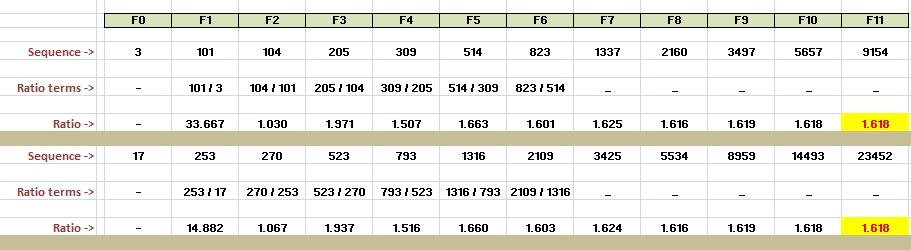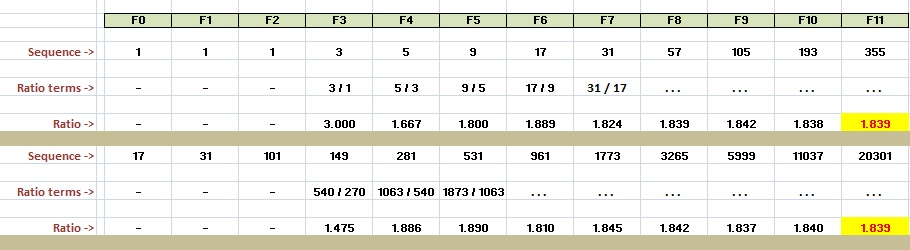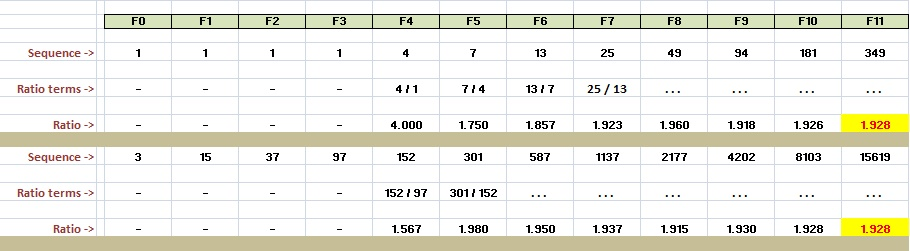# Fibonacci-like sequences and ratio of successive terms

Page 1 of 3   |   Page 2   |   Page 3

In this article, we explore some Fibonacci-like sequences. By that I don't mean sequences that look like Fibonacci himself, but sequences that are similar to the Fibonacci sequence.
Like most other investigations, the following one was a result of series of follow-up questions and attempt to find answer to those. As most of the readers are aware, Fibonacci sequence is a recurrence relation where a new term is generated by adding last 2 terms. The original Fibonacci sequence uses 1 and 1 as the first 2 terms (also called as seed values). The sequence looks like 1, 1, 2, 3, 5, 8, 13, etc. These terms are also denoted by F0, F1, F2, etc.

One of the notable features of this sequence is that the ratio of successive terms tends to golden ratio (1.618...)
That is, the ratios, 1/1, 2/1, 3/2, 5/3, 8/5, 13/5, etc. converge to 1.618..., which is also known as golden ratio.
The question that popped up in front of me was,
Does this special ratio only hold for seed values 1 & 1? Will I get the same ratio if I start with some other pair of numbers?"

Let's try to find out answer to this question using some sample sequences. Here are a couple of "Fibonacci" sequences, one using 3 and 101 as seed values, and the other using 17 and 253 (just some random values).We notice that for these sequences also, the ratio of consecutive terms tends to 1.618... (the golden ratio).
Conclusion : It does not matter which 2 numbers we start the sequence with, this ratio of successive terms always tends to 1.618... It may be simple to prove this, but I did not have much luck using a and b as starting terms, and trying to end up with golden ratio. May be some other approach is warranted, but not before we have some more adventure with similar sequences.
Conclusion : May be all sequences of this form are somehow "equivalent", meaning they share several similar properties (like the ratio). We may be able to form a "class" of such sequences. Further, we may be able to make some general statements about such sequences. Food for thought, to be dealt with later.
Further inquiry : Though ratio for all sequences of this kind converge to the same number, they do not converge at the same rate! It would be interesting to learn how the acceleration of convergence varies with seed values. We will save that for another time.

Follow up question : Is the ratio for all sequences of order 3 (i.e generated by adding last 3 terms) also the same? Let's find out.
Here are a couple of examples:Conclusion : For all sequences generated by adding last 3 terms, ratio of successive terms converges to another number 1.839... (we will find out the exact value soon).

What happens if we add last 4 terms?Conclusion : For last 4 terms, the ratio tends to 1.928...

Last 5 terms?Conclusion : For last 5 terms, the ratio tends to 1.961...
So is there a pattern emerging here? Let's tabulate the number of terms used for the recurrence, followed by the ratio.

Number of
successive terms
Ratio
2 1.618...
3 1.839...
4 1.928...
5 1.961...
... ...

We will need a computer to verify higher order sequences (order meaning number of terms we add), but looks like the ratios themselves converge to 2.
Thus, conclusion is: As the order of the sequences increase, the ratio of successive terms tends to 2.
We will prove this later in the article, but one of the questions that needs to be answered is what is the exact value of these ratios which we quoted approximately, viz. 1.618... , 1.839... , 1.928..., etc. Lets talk about it on the next page.

Page 1 of 3   |   Page 2   |   Page 3
 Quick Comment : * Name Email ( Your email id is safe with us! ) * Comments * Type the number of characters in the word "HUMAN"